# Laboratory Manual for Principles of General Chemistry: Exercise 9: Hard Water Analysis Pre-laboratory Assignment Flashcards

Set Details Share
created 11 years ago by momsaidno
1,845 views
updated 11 years ago by momsaidno
Subjects:
science, chemistry
Page to share:
Embed this setcancel
COPY
code changes based on your size selection
Size:
X

1

What cations are responsible for water hardness?

The cations responsible for water hardness are Ca2+ and Mg2+.

2

Experimental Procedure, Part A.1. Calculate the mass of disodium ethylenediaminetetraacetate (molar mass = 372.24 g/mol) required to prepare 250 mL of 0.010 M solution. Show the calculation. Express the mass to the correct number of significant figures.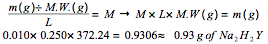3

Experimental Procedure, Part A.3. A 25.7-mL volume of prepared Na2H2Y solution titrates 25.0 mL of standard 0.0107 M Ca2+ solution to Eriochrome Black T endpoint. What is the molar concentration of the Na2H2Y solution?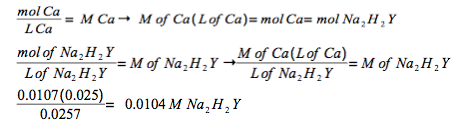4

Which hardening ion Ca2+ or Mg2+ , binds more tightly to (forms a stronger complex ion with) the Eriochrome Black T indicator used for today's analysis?

Periodic trends show that as you move down the rows the lattice energy decrease. Therefore, Mg should have a higher lattice energy then Ca and because of this Mg will form a stronger complex ion with the Black T indicator.

5

What is the color change at the endpoint?

Sky Blue.

6

Calculate the moles of hardening ions in the water sample.7

Assuming the hardness is due exclusively to CaCO3, express the hardness in mg CaCO3/L sample.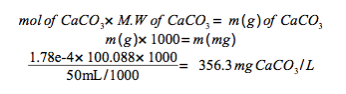8

What is the hardness concentration expressed in ppm CaCO3

1 mg CaCO3/L = 1 ppm
356.3 ppm of CaCO3

9

Classify the hardness of this water according to Table 9.1.

Very hard water

10

Determine the number of moles of hardening ions present in a 100-mL volume sample that has a hardness of 58 ppm CaCO3.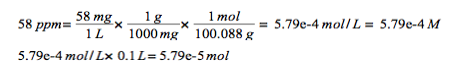11

Determine the number of moles of hardening ions present in a 100-mL volume sample that has a hardness of 58 ppm CaCO3.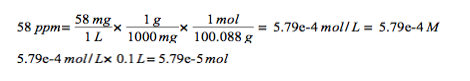12

What volume of the 0.100 M Na2H2Y is need to reach the Eriochorme Black T endpoint for the analysis of the solution.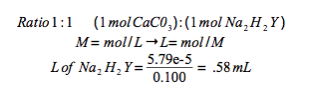13

Water hardness is also commonly expressed in units of grains/gallons, where 1 grain/gallon equals 17.1 ppm CaCO3. Express the hardness of this "slightly hard" water sample in grains/gallon.

58 ppm/ 17.1 ppm = 3.39 gpg